# Introduction to Linear Regression

The standard technique for measuring the relationship between one or more continuous independent variables and a continuous dependent variable is linear regression.

The basic idea of linear regression can be expressed simply. A linear regression is a line (or some more dimensional geometric thingy) that maps the independent variables to the best predicted value for the dependent variable.

Let's grab some data (we'll use the stuff from my book again to make it easy) and take a look at an example.

In :
import warnings
warnings.filterwarnings("ignore")

import pandas as pd
import seaborn as sns
import numpy as np
import matplotlib.pyplot as plt
import statsmodels.formula.api as sm
from mpl_toolkits.mplot3d import Axes3D
%matplotlib inline
%config InlineBackend.print_figure_kwargs = {'bbox_inches':None}


In :
df.head()

Out:
State Pop. In Millions for 2012 RoLScore elec_pros pol_plur free_expr assoc_org per_auto 2012GDP hprop hfisc hbiz hlab htra hinv
0 Albania 3.2 42.60 8 10 13 8 9 1.264810e+10 30 92.6 81.0 49.0 79.8 65
1 Argentina 41.1 51.94 11 15 14 11 13 4.755020e+11 15 64.3 60.1 47.4 67.6 40
2 Australia 22.7 73.28 12 15 16 12 15 1.532410e+12 90 66.4 95.5 83.5 86.2 80
3 Austria 8.4 73.15 12 15 16 12 15 3.947080e+11 90 51.1 73.6 80.4 86.8 85
4 Bangladesh 154.7 31.57 9 11 9 8 9 1.163550e+11 20 72.7 68.0 51.9 54.0 55
In :
sns.scatterplot(df["assoc_org"], df["pol_plur"])

Out:
<matplotlib.axes._subplots.AxesSubplot at 0x1142a8a58>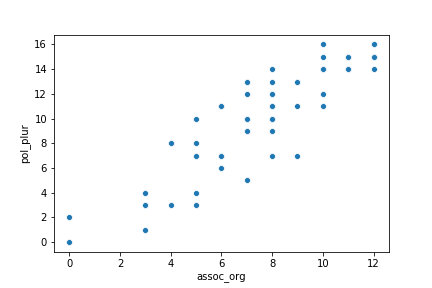It looks like there's a strong relationship between these variables (which is to be expected---they're measures, of, respectively, a country's level of political pluralism and its protections for freedom of association---obviously, countries that respect people's freedom to join groups are likely to get more diverse groups!)

The idea of linear regression is: suppose we draw a straight line, the regression line, through that graph in such a way as to minimize the distance between the line and the data points on there. (We actually minimize the squared distances, for mathematical reasons we won't bother with here.)

As you may recall from middle school, lines are described by an equation of the form

$$y=mx+b$$

which translates to "for everything on the x axis, multiply its value by m and then add b, and you get the corresponding value for the y axis."

The equation describing our regression line will represent our best prediction for values of y, given values of x. And the distances we minimize are the errors for that prediction, also known as the residuals.

In :
sns.regplot(df["assoc_org"], df["pol_plur"])

Out:
<matplotlib.axes._subplots.AxesSubplot at 0x1143772b0>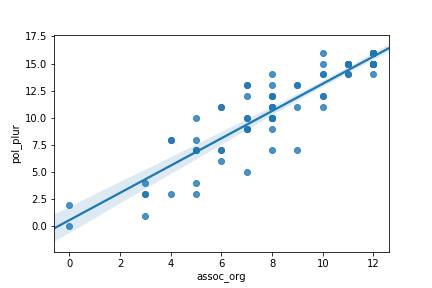We can see that our line carves right down the middle of our data.

We can also get the equation for the line in the form of a regression model.

In :
mod = sm.ols(formula='pol_plur ~ assoc_org', data=df)

res = mod.fit()

print(res.summary())

                            OLS Regression Results
==============================================================================
Dep. Variable:               pol_plur   R-squared:                       0.844
Method:                 Least Squares   F-statistic:                     481.8
Date:                Thu, 07 Feb 2019   Prob (F-statistic):           1.11e-37
Time:                        18:05:30   Log-Likelihood:                -174.68
No. Observations:                  91   AIC:                             353.4
Df Residuals:                      89   BIC:                             358.4
Df Model:                           1
Covariance Type:            nonrobust
==============================================================================
coef    std err          t      P>|t|      [0.025      0.975]
------------------------------------------------------------------------------
Intercept      0.5877      0.524      1.122      0.265      -0.453       1.629
assoc_org      1.2551      0.057     21.950      0.000       1.141       1.369
==============================================================================
Omnibus:                        4.087   Durbin-Watson:                   2.020
Prob(Omnibus):                  0.130   Jarque-Bera (JB):                3.869
Skew:                          -0.268   Prob(JB):                        0.145
Kurtosis:                       3.856   Cond. No.                         27.7
==============================================================================

Warnings:
 Standard Errors assume that the covariance matrix of the errors is correctly specified.


We'll talk about what most of this stuff means, but for now, just look down into the second sub-table where it says "Intercept" and "assoc_org". The first column of that table provides the coefficients. Where assoc_org is x and pol_plur is y, this is equivalent to saying that the regression line is

$$y = 1.2551x + 0.5877$$

and if you look at the chart, that looks about right. (Let's put a grid on there to make it clearer)

In :
with sns.axes_style("darkgrid"):
sns.regplot(df["assoc_org"], df["pol_plur"])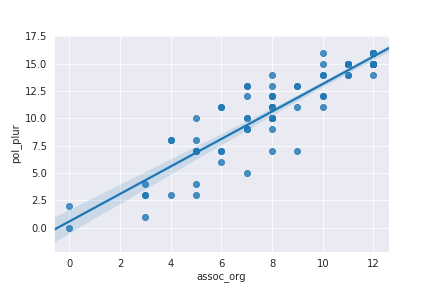You see how, for example, 10 on the x axis hits the regression line just a little bit above 12.5 (should be around 13) on the y axis. Our linear regression looks about right.

The string formula in the call to statsmodels, incidentally, is just the easiest way to compose a regression in Python. smf.ols(formula='pol_plur ~ assoc_org', data=df) means "run an ordinary least squares (ols) regression with pol_plur as the dependent, or y, variable, and assoc_org as the independent variable. Here's a description of how that formula system works. Generally you should use the fomula system with statsmodels regressions, in part because for some silly reason only when using the formulas does statsmodels calculate an intercept for our regression line.

Thinking about it visually for a second, our regression line actually describes the predicted value of our dependent variable for every value of our independent variable. The distance between the line and our plotted datapoints represents our residuals. With a bit more plotting work, we can visualize our residuals directly on the plot (don't worry about this code).

In :
def plot_with_residuals(x, y, fitted_model):
preds = fitted_model.predict()
fig, ax = plt.subplots()
x_sorted = np.sort(x)
y_sorted = y[np.argsort(x)]
preds_sorted = preds[np.argsort(x)]
ax.plot(x_sorted,preds_sorted)
ax.scatter(x_sorted,y_sorted)
ax.vlines(x_sorted,y_sorted,preds_sorted)

plot_with_residuals(df["assoc_org"], df["pol_plur"], res)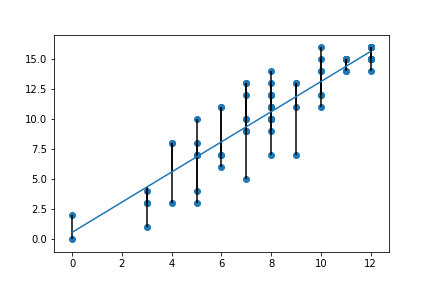The vertical lines there represent our residuals, although they don't give a full picture of them because some of them overlap. In order to see more of the residuals, we can add "jitter" to the scatterplot in order to make sure none are directly vertically on top of one another.

In :
def jitter(arr):
# swiped from https://stackoverflow.com/questions/8671808/matplotlib-avoiding-overlapping-datapoints-in-a-scatter-dot-beeswarm-plot
stdev = .01*(max(arr)-min(arr))
return arr + np.random.randn(len(arr)) * stdev

def plot_with_jitter(x, y, fitted_model):
preds = fitted_model.predict()
fig, ax = plt.subplots()
x_sorted = np.sort(x)
y_sorted = y[np.argsort(x)]
preds_sorted = preds[np.argsort(x)]
ax.plot(x_sorted,preds_sorted)
x_jittered = jitter(x_sorted)
ax.scatter(x_jittered,y_sorted)
ax.vlines(x_jittered,y_sorted,preds_sorted)

plot_with_jitter(df["assoc_org"], df["pol_plur"], res)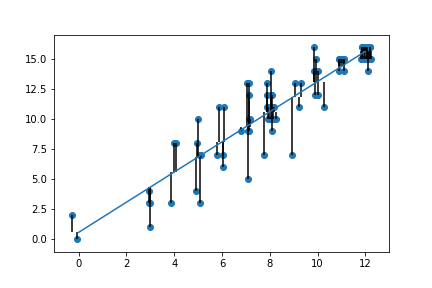The great thing about regression is that we don't have to limit ourselves to one variable. Here's something we can do:

In :
multi = sm.ols(formula='pol_plur ~ assoc_org + free_expr', data=df)
multires = multi.fit()
print(multires.summary())

                            OLS Regression Results
==============================================================================
Dep. Variable:               pol_plur   R-squared:                       0.868
Method:                 Least Squares   F-statistic:                     289.1
Date:                Thu, 07 Feb 2019   Prob (F-statistic):           2.07e-39
Time:                        18:05:30   Log-Likelihood:                -167.12
No. Observations:                  91   AIC:                             340.2
Df Residuals:                      88   BIC:                             347.8
Df Model:                           2
Covariance Type:            nonrobust
==============================================================================
coef    std err          t      P>|t|      [0.025      0.975]
------------------------------------------------------------------------------
Intercept     -0.7985      0.597     -1.338      0.184      -1.984       0.387
assoc_org      0.8328      0.118      7.032      0.000       0.597       1.068
free_expr      0.4086      0.103      3.986      0.000       0.205       0.612
==============================================================================
Omnibus:                       13.034   Durbin-Watson:                   1.833
Prob(Omnibus):                  0.001   Jarque-Bera (JB):               16.789
Skew:                          -0.693   Prob(JB):                     0.000226
Kurtosis:                       4.583   Cond. No.                         58.4
==============================================================================

Warnings:
 Standard Errors assume that the covariance matrix of the errors is correctly specified.


Here, the coefficients have the same meaning, they just describe a plane rather than a line, of the form $y = mx + nz + b$ where we've added z to for free_expr, and n= 0.4086.

This is the plane that minimizes squared distance between itself and the data we have, but now in three dimensions.

Note that the coefficients on assoc_org and the intercept have changed. This is to be expected. Conceptually, what this is doing is capturing the relationship between each of the independent variables x and z, if we held the other one constant.

If we want to be really fancy, we can even make a 3d plot of this regression plane. Though this is typically not very useful, as it's kind of hard to see useful things in 3d plots. Still, I'll do it to show off. (Again, no need to pay attention to this code. And I'm not even going to try to put in lines for the residuals here. If you want to get into 3d plotting, I recommend using a library that lets you work with interactive plots that you can drag around with the mouse and stuff---two good ones are bokeh and plotly.)

In :
def regplane3d(y, x1, x2, df):
fig = plt.figure(figsize=(10, 10))
# get the regression fit
model = sm.ols(formula='{} ~ {} + {}'.format(y, x1, x2), data = df)
fit = model.fit()
# lay out the range of x values for plotting and predicting
# here I'm going to switch notation conventions from regression (y = x1 + x2) to
# dimensions s.t. x1 and x2 become x and y, and y becomes z (the 3rd dimension)
x_surf = np.linspace(df[x1].min(), df[x1].max())
y_surf = np.linspace(df[x2].min(), df[x2].max())
x_surf, y_surf = np.meshgrid(x_surf, y_surf)
# predict over space of graph
synthetic_data = pd.DataFrame({x1: x_surf.ravel(), x2: y_surf.ravel()})
preds = fit.predict(exog=synthetic_data)
ax.plot_surface(x_surf, y_surf, preds.reshape(x_surf.shape),
rstride=1,
cstride=1,
color='None',
alpha = 0.4)
ax.scatter(df[x1], df[x2], df[y],
c='blue',
marker='o',
alpha=1)
return fig

In :
fig3d = regplane3d("pol_plur", "assoc_org", "free_expr", df)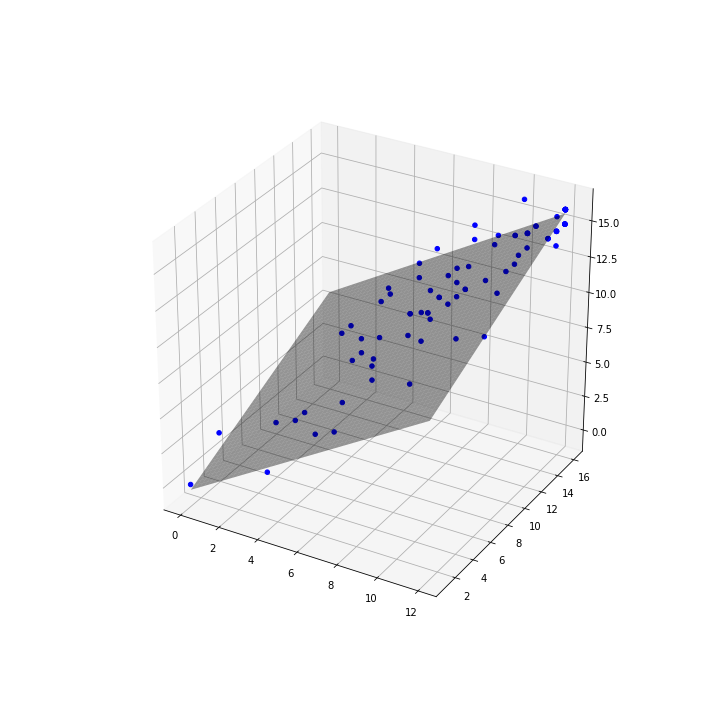Let's look at that summary again.

In :
print(multires.summary())

                            OLS Regression Results
==============================================================================
Dep. Variable:               pol_plur   R-squared:                       0.868
Method:                 Least Squares   F-statistic:                     289.1
Date:                Thu, 07 Feb 2019   Prob (F-statistic):           2.07e-39
Time:                        18:05:31   Log-Likelihood:                -167.12
No. Observations:                  91   AIC:                             340.2
Df Residuals:                      88   BIC:                             347.8
Df Model:                           2
Covariance Type:            nonrobust
==============================================================================
coef    std err          t      P>|t|      [0.025      0.975]
------------------------------------------------------------------------------
Intercept     -0.7985      0.597     -1.338      0.184      -1.984       0.387
assoc_org      0.8328      0.118      7.032      0.000       0.597       1.068
free_expr      0.4086      0.103      3.986      0.000       0.205       0.612
==============================================================================
Omnibus:                       13.034   Durbin-Watson:                   1.833
Prob(Omnibus):                  0.001   Jarque-Bera (JB):               16.789
Skew:                          -0.693   Prob(JB):                     0.000226
Kurtosis:                       4.583   Cond. No.                         58.4
==============================================================================

Warnings:
 Standard Errors assume that the covariance matrix of the errors is correctly specified.


Here's the least you need to know to make sense of this. The bottom box (Omnibus and Durbin-Watson and all that) are tests for the assumptions of regression; we'll talk about them in a later lesson.

The middle box has our coefficients, and then it has t-tests and p-values for the independent variables, which we can interpret more or less as usual. It also constructs 95% two-tailed confidence intervals, which are the last two columns. Here, our p values are for the two independent variables sufficiently low that statsmodels doesn't even bother displaying them. (They're not actually zero---they're never actually zero.) And, consistent with that, the confidence intervals don't include zero. (N.b., coefficients, p-values, and confidence intervals don't mean much on the intercept.)

In the first box, the most important things are the R-squared, which is a measure of the goodness of fit of our model, that is, the extent to which the model has enough information in it to account for all the variation in our dependent variable (as you should have seen in the readings). The thing about R-squared is that it always increases when you add more independent variables, and what counts as a good r-squared varies by problem domain, so it isn't all that useful except to compare two models with the same number (or very close) of independent variables and large differences in r-squared. An adjusted r-squared is a way to attempt to account for the number of variables in the model (i.e., reduce it as you add more independent variables. For more, the Minitab blog has a nice explanation of r-squared.

Another goodness of fit measure is the f-statistic which essentially is a test of the null hypothesis that all of the regression coefficients are equal to zero. Again, the Minitab blog has a nice explanation.

We'll be spending the next couple of weeks digging further into regression analysis---this is just the very basics, to get you an idea of the territory.